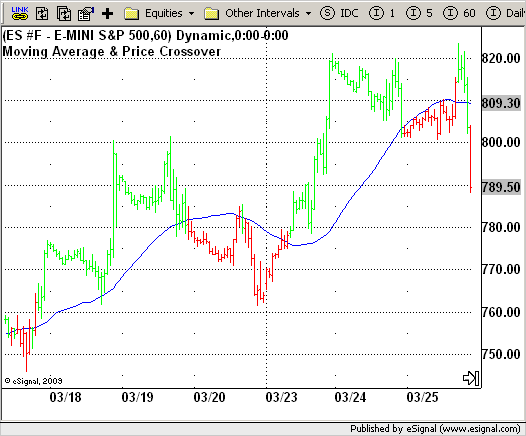# Moving Average & Price Crossover

ICE Data Services -

 strMA_Pcrsv.efs EFSLibrary - Discussion Board

File Name: strMA_Pcrsv.efs

Description:
Moving Average & Price Crossover

Formula Parameters:
Length : 40

Notes:
This is the almost simpliest strategy a trader can imagine. It is
based on moving average concept. A Moving Average is an indicator
that shows the average value of a security's price over a period of
time. When calculating a moving average, a mathematical analysis of
the security's average value over a predetermined time period is made.
As the security's price changes, its average price moves up or down.`/*********************************Provided By: eSignal (Copyright c eSignal), a division of Interactive Data Corporation. 2009. All rights reserved. This sample eSignal Formula Script (EFS) is for educational purposes only and may be modified and saved under a new file name. eSignal is not responsible for the functionality once modified. eSignal reserves the right to modify and overwrite this EFS file with each new release.Description: Moving Average & Price CrossoverVersion: 1.0 03/24/2009Formula Parameters: Default: Length 40Notes: This is the almost simpliest strategy a trader can imagine. It is based on moving average concept. A Moving Average is an indicator that shows the average value of a security's price over a period of time. When calculating a moving average, a mathematical analysis of the security's average value over a predetermined time period is made. As the security's price changes, its average price moves up or down. **********************************/var fpArray = new Array();var bInit = false;function preMain() { setPriceStudy(true); setStudyTitle("Moving Average & Price Crossover"); setCursorLabelName("SMA", 0); setColorPriceBars(true); setDefaultPriceBarColor(Color.black); var x=0; fpArray[x] = new FunctionParameter("Length", FunctionParameter.NUMBER); with(fpArray[x++]){ setLowerLimit(1); setDefault(40); }}var xSMA = null;function main(Length) {var nBarState = getBarState();var Close = close(-1);var nSMA = 0; if (nBarState == BARSTATE_ALLBARS) { if (Length == null) nLength = 40; } if (bInit == false) { xSMA = sma(Length); bInit = true; } nSMA = xSMA.getValue(-1); if (nSMA == null) return; if (getCurrentBarIndex() == 0) return; if(Close >= nSMA && !Strategy.isLong()) Strategy.doLong("Crossing Up", Strategy.MARKET, Strategy.THISBAR); if(Close < nSMA && !Strategy.isShort()) Strategy.doShort("Crossing Down", Strategy.MARKET, Strategy.THISBAR); if(Strategy.isLong()) setPriceBarColor(Color.lime); else if(Strategy.isShort()) setPriceBarColor(Color.red); return nSMA;}`